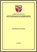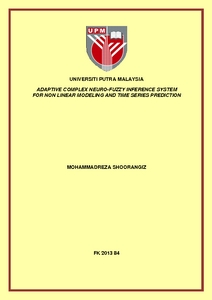# Adaptive complex neuro-fuzzy inference system for non linear modeling and time series prediction

## Citation

Shoorangiz, Mohammadreza (2013) Adaptive complex neuro-fuzzy inference system for non linear modeling and time series prediction. Masters thesis, Universiti Putra Malaysia.

## Abstract

Complex fuzzy logic is an extension to traditional fuzzy logic where truth values are extended to complex unit disk. It can be interpreted as a generalization of infinite-valued Type-1 fuzzy logic. Due to growth of truth values, complex fuzzy logic has better ability to deal with nonlinearities. Moreover, humans' brain works adaptively based on amplitude and phase which indicate that complex numbers are natural as well and can represent human brain more accurate in comparison with real valued numbers. Extension of this notion into fuzzy logic shows that complex fuzzy logic has better ability to model nonlinear system more accurately with smaller rule base comparing to type-1 fuzzy logic. At present, most of the researches are theoretical in nature, and practical works are presented to apply complex fuzzy logic for signal processing and time-series forecasting where the system is univariate. However, real world systems mostly have multiple input and therefore complex fuzzy logic can be extended for modeling of these kind of systems. To this end, in this study a machine learning architecture based on complex fuzzy logic has been developed. This structure is developed to deal with nonlinear system modeling as well as time-series forecasting with multiple inputs. The development sequence of such method can be divided into two parts: Developing network structure to employ complex fuzzy logic and proposing learning algorithm to train the system. For the first part,a structure based on a well-known adaptive network fuzzy inference system has been proposed and also Takagi-Sugeno inferential system has been modified. Second part has been done by proposing a novel learning rule containing genetic algorithm, Levenberg-Marquardt technique and least square estimation. In this regard, genetic algorithm generates different initial conditions of premise parameters to and the best one. Furthermore, Levenberg-Marquardt technique and least square estimation are employed to one tune the premise and consequent parameters, respectively. To this end, a closed form derivative solution of error with respect to premise parameters has been derived. Afterward, the performance of proposed method has been evaluated using five different systems consisting of nonlinear function modelings, nonlinear dynamic modeling, chaotic dynamic prediction and sunspot dataset forecasting. The procedure of data selection for training tasks of these experiments have been taken from literature. This leads to conduct the experiments in the same condition as previous works and therefore be reasonable to compare with their results. The performance of the proposed method is then compared with other works in literature. The first experiment is two dimensional Sinc function in which the proposed method with 4 rules has root mean square error of 0.017 while the root mean square error of adaptive network fuzzy inference system method is 0.074. This shows that proposed method has better ability to model this nonlinear system. The second experiment used proposed method to model a three input nonlinear function. The number of rules for this experiment is same for proposed method and adaptive network fuzzy inference system. However, the average percentage error of proposed method is 0.193% which is much lower than 1.066% for previous method. A nonlinear dynamic system has been modeled in the third experiment. The proposed method has root mean square error of 0.018 with 4 number of rules. In this regard, the error value is less than previous approaches while other approaches have same or higher number of rules. The other two experiments are done to predict two well known time series. Mackey-Glass is the first time series which is known as chaotic and aperiodic. Proposed method with 8 number of rules is able to predict this dataset with root mean square of 0.0007 and non dimensional error index of 0.0031 which is comparable with previous works in terms of error measure values and number of rules. The second time series database is annual sunspot dataset which is a natural and physical phenomena. The proposed method is able to predict this dataset with 8 number of rules. The values of error measures for this experiments are 2:2 10-3 for mean squared error and 0.209 for non dimensional error index. The performance of proposed method in terms of error measures is better than previous approaches. The comparisons of performances in terms of number of rules and error measures for performed experiments between proposed method and previous approaches reveal the capability and effectiveness of proposed method in modeling nonlinear systems and forecasting of time series.Preview
PDF
FK 2013 84RR.pdfView Item